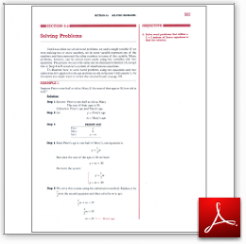Algebra Math Book - Introductory Algebra Thank you for your continual support.

 The power of mathematics rests in the logic of thinking.ID: Sec8-4
Description: Age Problems-Coins-Geometry
Price: 1.99

# Algebra Math book - Introductory Algebra - Chapter 8 - Section 4 - Solving Problems

## Section 8.4 - Objectives

4.  Solve word problems that utilize a 2 x 2 system of linear equations to find the solution.

Navigate to
Previous Section or Next Section
or Chapter 8 Details or Other Chapters

This section of my algebra math book, Introductory Algebra, also includes in the download:

• Cover Sheet